ChemTalk

# Henderson-Hasselbalch Equation## Core Concepts – Henderson-Hasselbalch Equation

In this tutorial, you will learn what the Henderson-Hasselbalch equation is, how to use the equation to determine the pH of a buffer, how to derive the equation, and how to solve some example problems

## What is the Henderson Hasselbalch Equation?

The Henderson-Hasselbalch equation is used to determine the pH of a buffer system.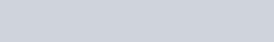The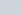is the acid dissociation constant, and pKa is the negative log of. [Conjugate Base] is also commonly written as [A-]. And [Weak Acid] may be seen as [HA]. These values refer to the equilibrium concentration.

If you have the same concentration of acid and conjugate base, then the pH will be the same as the pKa because the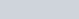.

If [HA] = [A], then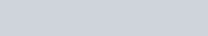Phosphoric acid buffer solution that can be analyzed using the Henderson-Hasselbalch Equation

## Derivation of the Henderson-Hasselbalch Equation

The Henderson-Hasselbalch equation can be derived from theof a weak acid. Here we will assume we have a weak acid, HA, and its conjugate base, A. In water the following dissociation occurs: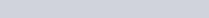Therefore, the acid dissociation constant is defined as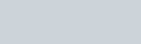Which can be rearranged to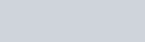Then take the log of both sides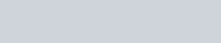Multiply by -1 so that in the next step we can substitute in for pH.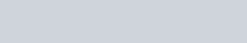Based on the definition of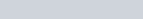we can substitute into our equation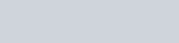And then use logarithm rules to simplify the right side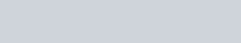Knowing that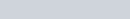, we can substitute that into our previous equation to arrive at the Henderson-Hasselbalch equation## Example Problems of Henderson Hasselbalch Equation

#### Determining Buffer Ratio

Suppose we are making a buffer solution of ammonium (NH4+) and ammonia (NH3). In this example, ammonium is our weak acid and ammonia is the conjugate base. The reaction is as follows.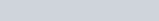If the pKa of ammonium is 9.3 and we want a pH of 10. What ratio of ammonia to ammonium should you add to the solution?

The problem asks for a ratio, so we are looking for the value of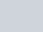, or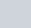for this specific buffer solution. To solve the problem, start by plugging in the known values– pH and pKa.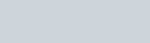Subtract 9.3 from each side to simplify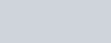The ratio we are looking for is within the logarithmic term. To get rid of the log we can use logarithmic rules to simplify.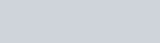Therefore, the ratio is about 5 times the amount of ammonia as ammonium to get a buffer solution with a pH of 10.

#### Determining pH

Use the Henderson-Hasselbalch equation to determine the pH of a buffer solution made up of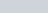CH3COO and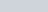CH3COOH. The pKa of acetic acid (CH3COOH) is 4.75.

To solve this example problem, we want to start with the Henderson-Hasselbalch equation since we are looking at a buffer solution of a weak acid and conjugate base.Then plug the known values into our equation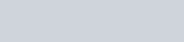Then simplify and solve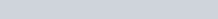Our buffer solution of acetic acid has a pH of 4.63.

## Limitations of the Henderson-Hasselbalch Equation

1. When using the Henderson-Hasselbalch to make a buffer solution the ratio ofshould be close to one. This makes the most stable solution. When the ratio moves far away from one, the capacity to buffer the solution decreases as a small disturbance changes the pH dramatically.
2. This pH equation does not account for the self-dissociation of water. At very low concentrations of buffer, water can contribute to the pH
3. If an acid can easily dissociate more than one hydronium ion (H+) the equation does not account for that and may not work well.
4. Practically when making specific pH buffers, it is important to have the pKa be close to the target pH to have the biggest range of buffering capability.

## Fun Facts:

• The equation takes its name after Lawrence Joseph Henderson and Karl Albert Hasselbalch. Henderson made the original equation in 1908. Then in 1917 Hasselbalch put the formula into logarithmic terms and the way it is most seen today.
• The Henderson-Hasselbalch equation has important biological relevance. Many biological samples require a specific pH. So, when biologists prepare samples, the Henderson-Hasselbalch equation ensures a pH that helps proteins and other pH sensitive molecules maintain their structure.
• A similar equation can calculate the pOH of a buffer system.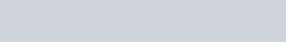The [Conjugate Acid] is also referred to as [HB+]. And the [Weak Base] is also referred to as [B]. Try checking your understanding by going through the derivation for the pOH version of the equation.

## Henderson-Hasselbalch Equation Practice Problems

Problem 1

The organic acid acetylacetone has a pKa of 8.99, with the following dissociation reaction: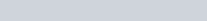In a buffer solution with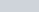of C5H7O2 andof C5H8O2, what is the final pH?

Problem 2

Buffer A is an ammonia solution with an conjugate base-acid ratio of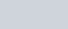. Buffer B is an acetic acid solution with an conjugate base-acid ratio of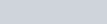. Which is more acidic?

1: 8.81

2: Buffer B